Courses

# Ex 6.2 NCERT Solutions- Lines and Angles Class 9 Notes | EduRev

## Class 9 : Ex 6.2 NCERT Solutions- Lines and Angles Class 9 Notes | EduRev

The document Ex 6.2 NCERT Solutions- Lines and Angles Class 9 Notes | EduRev is a part of the Class 9 Course Mathematics (Maths) Class 9.
All you need of Class 9 at this link: Class 9

Exercise 6.2

Q.1. In the following figure, find the values of x and y and then show that AB CD.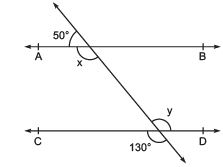Solution:
We know that a linear pair is equal to 180°.
So, x+50° = 180°
∴ x = 130°
We also know that vertically opposite angles are equal.
So, y = 130°
In two parallel lines, the alternate interior angles are equal. In this,
x = y = 130°
This proves that alternate interior angles are equal and so, AB CD.

Q.2. In the following Figure, if AB CD, CD EF and y : z = 3 : 7, find x.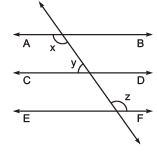Solution:
It is known that ABCD and CDEF
As the angles on the same side of a transversal line sums up to 180°,
x + y = 180° —–(i)
Also,
O = z (Since they are corresponding angles)
and, y +O = 180° (Since they are a linear pair)
So, y+z = 180°
Now, let y = 3w and hence, z = 7w (As y : z = 3 : 7)
∴ 3w+7w = 180°
Or, 10 w = 180°
So, w = 18°
Now, y = 3×18° = 54°
and, z = 7×18° = 126°
Now, angle x can be calculated from equation (i)
x+y = 180°
Or, x+54° = 180°
∴ x = 126°

Q.3. In the following figure, if AB CD, EF ⊥ CD and GED = 126°, find AGE, GEF and FGE.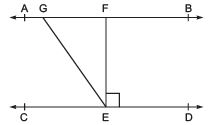Solution:
Since AB CD, GE is a transversal.
It is given that GED = 126°
So, GED = AGE = 126° (As they are alternate interior angles)
Also,
GED = GEF +FED
As EF⊥ CD, FED = 90°
∴ GED = GEF+90°
Or, GEF = 126° – 90° = 36°
Again, FGE +GED = 180° (Transversal)
Putting the value of GED = 126° we get,
FGE = 54°
So,
AGE = 126°
GEF = 36° and
FGE = 54°

Q.4. In the following figure, if PQ ST, PQR = 110° and RST = 130°, find QRS.
[Hint : Draw a line parallel to ST through point R.]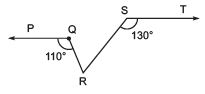Solution: First, construct a line XY parallel to PQ.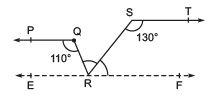We know that the angles on the same side of transversal is equal to 180°.
So, PQR+QRX = 180°
Or, QRX = 180°-110°
∴ QRX = 70°
Similarly,
RST +SRY = 180°
Or, SRY = 180°- 130°
∴ SRY = 50°
Now, for the linear pairs on the line XY-
QRX+QRS+SRY = 180°
Putting their respective values, we get,
QRS = 180° – 70° – 50°
Hence, QRS = 60°

Q.5. In the following figure, if AB CD, APQ = 50° and PRD = 127°, find x and y.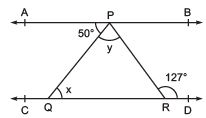Solution:
From the diagram,
APQ = PQR (Alternate interior angles)
Now, putting the value of APQ = 50° and PQR = x we get,
x = 50°
Also,
APR = PRD (Alternate interior angles)
Or, APR = 127° (As it is given that PRD = 127°)
We know that
APR = APQ+QPR
Now, putting values of QPR = y and APR = 127° we get,
127° = 50°+ y
Or, y = 77°
Thus, the values of x and y are calculated as:
x = 50° and y = 77°

Q.6. In the following figure, PQ and RS are two mirrors placed parallel to each other. An incident ray AB strikes the mirror PQ at B, the reflected ray moves along the path BC and strikes the mirror RS at C and again reflects back along CD. Prove that AB CD.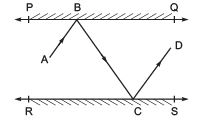Solution:

First, draw two lines BE and CF such that BE ⊥ PQ and CF ⊥ RS.

Now, since PQ RS,

So, BE CF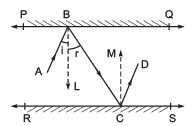We know that,
Angle of incidence = Angle of reflection (By the law of reflection)
So,
1 = 2 and
3 = 4
We also know that alternate interior angles are equal. Here, BE ⊥ CF and the transversal line BC cuts them at B and C
So, 2 = 3 (As they are alternate interior angles)
Now, 1 +2 = 3 +4
Or, ABC = DCB
So, AB CD alternate interior angles are equal)

Exercise 6.3

Q.1. In the adjoining figure, sides QP and RQ of ΔPQR are produced to points S and T respectively. If ∠ SPR = 135º and ∠ PQT = 110º, find ∠ PRQ.
Solution: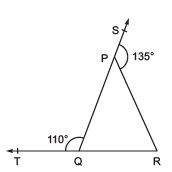It is given the TQR is a straight line and so, the linear pairs (i.e. TQP and PQR) will add up to 180°
So, TQP +PQR = 180°
Now, putting the value of TQP = 110° we get,
PQR = 70°
Consider the ΔPQR,

Here, the side QP is extended to S and so, SPR forms the exterior angle.
Thus, SPR (SPR = 135°) is equal to the sum of interior opposite angles. (Triangle property)
Or, PQR +PRQ = 135°

Now, putting the value of PQR = 70° we get,
PRQ = 135°-70°

Hence, PRQ = 65°

Q.2. In Fig. X = 62°, XYZ = 54°. If YO and ZO are the bisectors of XYZ and XZY respectively of Δ XYZ, find OZY and YOZ.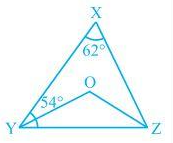Solution:
We know that the sum of the interior angles of the triangle.
So, X +XYZ +XZY = 180°
Putting the values as given in the question we get,
62°+54° +XZY = 180°
Or, XZY = 64°
Now, we know that ZO is the bisector so,
OZY = ½ XZY
∴ OZY = 32°
Similarly, YO is a bisector and so,
OYZ = ½ XYZ
Or, OYZ = 27° (As XYZ = 54°)
Now, as the sum of the interior angles of the triangle,
OZY +OYZ +O = 180°
Putting their respective values, we get,
O = 180°-32°-27°
Hence, O = 121°

Q.3. In the following figure, if AB DE, BAC = 35° and CDE = 53°, find DCE.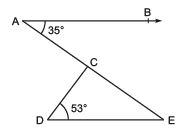Solution:
We know that AE is a transversal since AB DE
Here BAC and AED are alternate interior angles.
Hence, BAC = AED
It is given that BAC = 35°
AED = 35°
Now consider the triangle CDE. We know that the sum of the interior angles of a triangle is 180°.
∴ DCE+CED+CDE = 180°
Putting the values, we get
DCE+35°+53° = 180°

Hence, DCE = 92°

Q.4. In the figure,  if lines PQ and RS intersect at point T, such that PRT = 40°, RPT = 95° and TSQ = 75°, find SQT.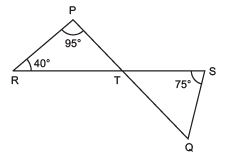Solution:
Consider triangle PRT.
PRT +RPT + PTR = 180°
So, PTR = 45°
Now PTR will be equal to STQ as they are vertically opposite angles.
So, PTR = STQ = 45°
Again, in triangle STQ,
TSQ +PTR + SQT = 180°
Solving this we get,
SQT = 60°

Q.5. In the adjoining figure, if PQ ⊥ PS, PQ SR, SQR = 28° and QRT = 65°, then find the values of x and y.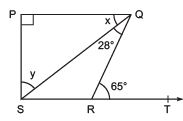Solution:
x +SQR = QRT (As they are alternate angles since QR is transversal)
So, x+28° = 65°
∴ x = 37°
It is also known that alternate interior angles are same and so,
QSR = x = 37°
Also, Now,
QRS +QRT = 180° (As they are a Linear pair)
Or, QRS+65° = 180°
So, QRS = 115°
Now, we know that the sum of the angles in a quadrilateral is 360°. So,
P +Q+R+S = 360°
Putting their respective values, we get,
S = 360°-90°-65°-115°
In Δ SPQ
∠SPQ + x + y = 1800
900 + 370 + y = 1800
y = 1800 – 1270 = 530
Hence, y = 53°

Q.6. In the adjoining figure, the side QR of ΔPQR is produced to a point S. If the bisectors of PQR and PRS meet at point T, then prove that QTR = ½ QPR.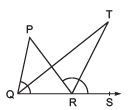Solution:
Consider the ΔPQR. PRS is the exterior angle and QPR and PQR are interior angles.
So, PRS = QPR+PQR (According to triangle property)
Or, PRS -PQR = QPR ———–(i)
Now, consider the ΔQRT,
TRS = TQR+QTR
Or, QTR = TRS-TQR
We know that QT and RT bisect PQR and PRS respectively.
So, PRS = 2 TRS and PQR = 2TQR
Now, QTR = ½ PRS – ½PQR
Or, QTR = ½ (PRS -PQR)
From (i) we know that PRS -PQR = QPR
So, QTR = ½ QPR (hence proved).

Offer running on EduRev: Apply code STAYHOME200 to get INR 200 off on our premium plan EduRev Infinity!

## Mathematics (Maths) Class 9

46 videos|323 docs|108 tests

,

,

,

,

,

,

,

,

,

,

,

,

,

,

,

,

,

,

,

,

,

;